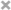## 网易算法工程师岗编程题

输入两行数据，第一行是两个整数：路灯数目n(1<=n<=1000)，街道长度(1<=l<=pow(10, 9))。第二行有n个整数ai(0<=ai<=l)，表示路灯坐标，多个路灯可以在同一个地方，也可以安放在终止点位置。


5 5 4
5 2
4 7
3 1
3 2
2 5


4


Hint:

花两个小时复习第三门考试拿两分，两个小时复习第四门考试拿一分，这样总平均成绩为(5+4+5+4+2)/4 = 4


#include <iostream>
#include <vector>
#include <algorithm>
#include <numeric>

using namespace std;

int main(int argc, const char * argv[]) {

int n, r, avg;
cin >> n >> r >> avg;

vector<int> A(n);
vector<int> B(n);

for(int i = 0; i < n; i++){
int tmpAi, tmpBi;
cin >> tmpAi >> tmpBi;
A[i] = tmpAi;
B[i] = tmpBi;
}

vector<size_t> indexes(n);
size_t nn(0);
generate(begin(indexes), end(indexes), [&]{return nn++;});
sort(begin(indexes), end(indexes), [&](int i1, int i2){return B[i1] < B[i2];});
vector<int> sortedA(n);
vector<int> sortedB(n);
for(int i = 0; i < n; i++){
sortedB[i] = B[indexes[i]];
sortedA[i] = A[indexes[i]];
}

int sum = accumulate(sortedA.begin(), sortedA.end(), 0);
int time = 0;
int i = 0;
while((sum < avg*n) && (i < n)){
while((sortedA[i] + addScores) < 5 && sum < avg*n){
sum = sum + 1;
time = time + sortedB[i];
}
++i;
}

cout << time << endl;

return 0;
}


输入两行数据，第一行是两个整数：路灯数目n(1<=n<=1000)，街道长度(1<=l<=pow(10, 9))。第二行有n个整数ai(0<=ai<=l)，表示路灯坐标，多个路灯可以在同一个地方，也可以安放在终止点位置。


7 15
15 5 3 7 9 14 0


2.5


#include <iostream>
#include <vector>

using namespace std;

int main(int argc, const char * argv[]) {

int numBulb, distance;
cin >> numBulb >> distance;

vector<int> looc(numBulb);

for(int i = 0; i < numBulb; i++){
int tmp;
cin >> tmp;
looc[i] = tmp;
}

sort(looc.begin(), looc.end(), less<int>());
int maxDis = 0;
for(int i = 0; i < numBulb; i++){
int tmp = looc[i+1]-looc[i];
if(tmp > maxDis)
maxDis = tmp;
}

cout << maxDis/2.0 << endl;

return 0;
}


## 大众点评数据挖掘岗综合题

•  设H1表示A部门，H2表示B部门，P(H1) = P(H2) = 0.5, P(E H1) = 30/54, P(E H2) = 12/54;
•  设E表示女生，则问题转成求P(H1 E)；
•  P(E) = P(E H1)P(H1) + P(E H2)P(H2) = 7/18;
• 根据条件概率公式有：
•  P(H1 E) = P(H1)*P(E H1)/P(E) = 0.5*(30/54)/(7/18) = 0.714

## 京东算法岗综合题

You are the best
123
To be NO.1
Yes
Thank you very much
%%%


You1234are1234the1234best
ToYesbeYesNO.1
Thank%%%you%%%very%%%much


#include <iostream>
using namespace std;

char* replace_blank(char str[], char strB[], int len, int lenB)
{
if(str == NULL || len <= 0) {
return NULL;
}

int i = 0, numberBlanks = 0; // cnt为整个字符串的空格个数，size为字符串字符个数
while(str[i] != '\0') {
if(str[i] == ' ') {
++numberBlanks;
}
++i;
}

for(i = len-1; i >= 0; --i) {
if(str[i] != ' ') {
str[i+numberBlanks*(lenB - 1)] = str[i];
// 测试输出
//char tt = str[i];
//int tmp = i+numberBlanks*(lenB - 1);
//cout << tmp << ": " << tt << endl;
}
else {
for(int j = 0; j < lenB; j++){
str[i+numberBlanks*(lenB - 1) - j] = strB[lenB -1 - j];
// 测试输出
//char tt = strB[lenB -1 - j];
//int tmp = i+numberBlanks*(lenB - 1) - j;
//cout << tmp << ": " << tt << endl;
}
--numberBlanks;
}
}
return str;
}

int main(int argc, const char * argv[]) {

string inputA, inputB;
getline(cin, inputA);
getline(cin, inputB);
int lenA = (int)inputA.length();
int lenB = (int)inputB.length();
char strA[lenA];
char insertedB[lenB];
strcpy(strA, inputA.c_str());
strcpy(insertedB, inputB.c_str());
char* afterInserted = replace_blank(strA, insertedB, lenA, lenB);
cout << afterInserted << endl;

return 0;
}


输入4个数字，分别表示四个小球距离地面的高度，所有输入的数字都为整数，范围不能超过整数所能表示的最大值。


输出为4个小球经过的总长度


100 90 80 70


996


int ballDistance(int h){
int sumH = h;
int halfH = (int)h/2.0;
while(halfH != 0){
sumH = sumH + 2*halfH;
halfH = (int)halfH/2.0;
}
return sumH;
}

int main(int argc, const char * argv[]) {

int t1, t2, t3, t4;
cin >> t1 >> t2 >> t3 >> t4;
int sum = ballDistance(t1) + ballDistance(t2)  + ballDistance(t3) + ballDistance(t4);
cout << sum << endl;
return 0;
}


## Nice笔试题

9月10号晚nice在西电宣讲，随后进行了笔试，时间1个小时，下面是当晚回来后做的笔试回忆及总结。

In the OSI model it’s less defined because encryption is in Layer 6 and session control is in Layer 5. HTTPS (layer 7) uses SSL and SSL (Layers 5/6) uses TCP (Layer 4). SSL negotiation actually starts in Layer 5 and the encrypted tunneling kicks in after the SSL handshake is successful, so I would call SSL an OSI Layer 5 protocol.

According to the AIO, 6th edition (p. 531), SSL and TLS work at the transport layer of the OSI model.

According to CISSP for Dummies (p. 259), “SSL operates at the Transport Layer (Layer 4) of the OSI model…”

char* p = (char*)malloc(100);


struct listNode{
int value;
listNode* next;
};

listNode* merge(listNode* L1, listNode* L2){
if(L1 == NULL || L2 == NULL)
return ____? _____:______
}
......(后面还很多）


listNode* merge(listNode* L1, listNode* L2){
if(L1 == NULL)
return L2;
else if(L2 == NULL)
return L1;
}


listNode* merge(listNode* pHead1, listNode* pHead2){
}else{
}
}


void test(){
long i;
long a;
for (i = 0; i <= 17, ++1){
a[i] = 0;
fprint("%d", a[i]);
}
}


C++参考答案：

struct listNode{
int value;
listNode* Next;
}

listNode* preNode = NULL;  //前一个结点
while(pNode != NULL){
listNode* pNext = pNode->Next;  //下一个结点
while(pNext == NULL)
pNext->Next = preNode;
preNode = pNode;
pNode = pNext;
}
}